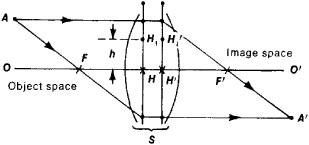# Cardinal Points of an Optical System

The following article is from The Great Soviet Encyclopedia (1979). It might be outdated or ideologically biased.

## Cardinal Points of an Optical System

the points on the optical axis OO’ (see Figure 1) of a centered optical system that can be used to construct the image of an arbitrary point in space for objects in the paraxial region, which is the region around the axis of symmetry of the system where a point is represented by a point, a straight line by a straight line, and a plane by a plane.Figure 1. Position of the image A’ of an arbitrary point A projected by an optical system S can be found if the cardinal points F, F’, H, and H’ of the system are known: a ray passing through the front focus F is directed by the system parallel to its optical axis OO’, and a ray that is incident upon the system parallel to the axis OO’ is directed through the back focus F’ after refraction

There are four cardinal points in an optical system: the front and back foci F and F’ and the front and back principal points H and H’. The back focus is the image of an infinitely remote point located on the optical axis in the object space, and the front focus is the image in the object space of an infinitely remote point in the image space. The principal points are the points of intersection with the optical axis of the principal planes, which are the planes for which the optical system S produces full-size mutual images (every point H1 located in the principal plane HH1 at a distance h from the axis OO’ appears in the other principal plane H’ H’1 as the point H’1 at the same distance h from the axis as point H1).

The distance from point H to point F is called the front focal distance (negative in the figure), and the distance from point H’ to point F’ is called the back focal distance (positive in the figure).

The construction of an image A’ of an arbitrary point A for a centered optical system using the points F, H, H’, and F’ is shown in Figure 1.

### REFERENCE

Tudorovskii, A. I. Teoriia opticheskikh priborov, 2nd ed. [part] 1. Moscow-Leningrad, 1948. Page 265. G. G. Sliusarev